# Dynamic nature of equilibrium

Equilibrium: Dynamic nature of equilibrium

In this type of reaction, no observable net change in the system occurs. Reactions that continue to proceed in the forward and reverse reaction dynamically, however, there is no net change in the amount of product or the starting material. In a dynamic equilibrium, there is motion despite there being no net change. Dynamic equilibrium is also called steady state. On the other hand, the static equilibrium state is one where there is no motion at the steady state. It is also called mechanical equilibrium which occurs when the particles are at rest and there is no movement of vibration, translation or rotation of reactants and products. The static steady state is viewable at the macroscopic level. In an irreversible reaction, the reaction proceeds until all the reactants are used to make products. Once the reactants are limiting, the reaction stops, and static equilibrium is achieved.

For example, an irreversible reaction such as the conversion of graphite to diamond enters a static equilibrium where all the atoms of carbon are bonded together in covalent bonds and their movement is restricted. The equilibrium constant cannot show if the reaction is static or dynamic since it only presents the ration between reactants and products. Therefore, the rate of the reactions play an important role in defining the reaction to be dynamic or not. Reaction order and rates are determined experimentally however, the stoichiometric factors of the balanced equation may be used to predict reaction rates.

Dynamic reactions are reversible reactions. In the early stage of a reversible reaction, the forward reaction proceeds more quickly than the reverse reaction until the equilibrium state is reached where the forward and reverse rates are equal. Reaction rates are graphed by plotting the concentration in moles per litre by the time in seconds as shown in the figures below.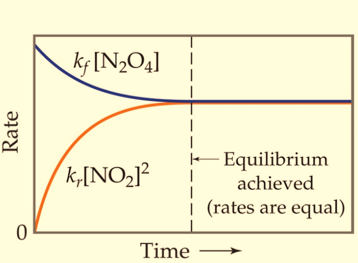Figure 1: Reactions where the rates of forward and backward reactions are equal.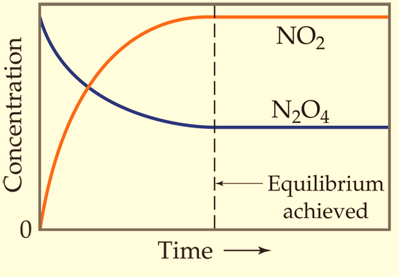Figure 2: Reactions where the concentration of forward and backward constituents is equal

The concentrations and reaction rate (fewer collisions, less component) of reactants decreases over time as the concentrations and reaction rate of products increases (more collisions, more component) over time until the rates are equal,and the concentrations of each component reach a constant value.

Consider the reaction between hydrogen gas and carbon monoxide: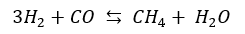Here, as the two reactants are used up, the forward rate of reaction decreases over time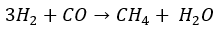And, as the two products increase in quantity, the backward rate of reaction increases over time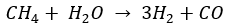Soon, the rate of forward and backward reaction becomes equal, where the concentration of all the constituents is equal. The two reactions continue to run simultaneously but there is no more visible or measurable change in the system. This is the point where dynamic equilibrium is established in the system.